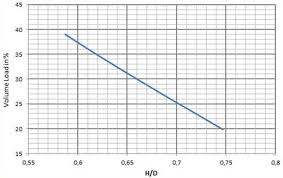## How to Calculate and Solve for Shaft Power | Ball Mill LengthThe image above represents shaft power | ball mill length.

To compute for shaft power | ball mill length, six essential parameters are needed and these parameters are Value of C, Volume Load Percentage (J), % Critical Speed (Vcr), Bulk Density (s.g), Mill Length (L) and Mill Internal Diameter (D).

The formula for calculating shaft power | ball mill length:

P = 7.33 x C X J X Vcr x (1 – 0.937) x [1 – 0.1/29 – 10Vcr] x s.g. x L x D2.3

Where:

P = Shaft Power | Ball Mill Length
Vcr = % Critical Speed
sg = Bulk Density
L = Mill Length
D = Mill Internal Diameter

Let’s solve an example;
Find the shaft power | ball mill length when the volume load percentage is 8, the %critical speed is 10, the bulk density is 3. the mill length is 14 and the mill internal diameter is 16.

This implies that;

J = Volume Load Percentage = 8
Vcr = % Critical Speed = 10
sg = Bulk Density = 3
L = Mill Length = 14
D = Mill Internal Diameter = 16

P = 7.33 x C X J X Vcr x (1 – 0.937) x [1 – 0.1/29 – 10Vcr] x s.g. x L x D2.3
P = 7.33 x 1 x 8 x 10 x (1 – 0.937) x [1 – 0.1/29 – 10(10)] x 3 x 14 x 162.3
P = 36.94 x [1 – 0.1/2-91] x 3 x 14 x 588.133
P = 36.94 x [1 – 0.1/4.038e-28] x 24701.609
P = 36.94 x [1 – 2.47e+26] x 24701.609
P = 36.94 x -2.475e+26 x 24701.609
P = -2.259

Therefore, the shaft power | ball mill length is – 2.259 W.